# ISEE Math Practice Test: Plugging In

### Test Information16 questions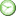0 minutes

Take more free ISEE Mathematics Achievement Practice Tests available from crackssat.com.

1. At a charity fund-raiser, 200 people each donated x dollars. In terms of x, what was the total number of dollars that was donated?

• A.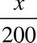• B. 200x
• C.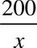• D. 200 + x

2. If 10 magazines cost d dollars, how many magazines can be purchased for 3 dollars?

• A.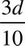• B. 30d
• C.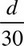• D.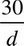3. The zoo has four times as many monkeys as lions. There are four more lions than there are zebras at the zoo. If z represents the number of zebras in the zoo, then in terms of z, how many monkeys are there in the zoo?

• A. 4z
• B. z + 4
• C. 4z + 16
• D. 4z + 4

4. There were 6 pairs of earrings sold at a price of y dollars each. In terms of y, what is the total amount of money for which these earrings were sold?

• A. 6 + y
• B. 6y
• C. 6y
• D. 6 + 6y

5. If p pieces of candy costs c cents, 10 pieces of candy will cost

• A.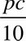cents
• B.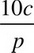cents
• C.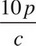cents
• D. 10pc cents

6. If J is an odd integer, which of the following must be true?

• A. (J ÷ 3) > 1
• B. (J - 2) is a positive integer.
• C. 2 × J is an even integer.
• D. J > 0

7. On Monday, Sharon ate one-half of a fruit tart. On Tuesday, Sharon then ate one-fourth of what was left of the tart. What fraction of the tart did Sharon eat on Monday and Tuesday?

• A.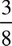• B.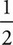• C.• D.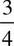8. The price of a suit is reduced by 20%, and then the resulting price is reduced by another 10%. The final price is what percent off of the original price?

• A. 20%
• B. 25%
• C. 28%
• D. 30%

9. If m is an even integer, n is an odd integer, and p is the product of m and n, which of the following is always true?

• A. p is a fraction.
• B. p is an odd integer.
• C. p is divisible by 2.
• D. p is greater than zero.

10. If p is an odd integer, which of the following must be an odd integer?

• A. p2 + 3
• B. 2p + 1
• C. p ÷ 3
• D. p - 3

11. If m is the sum of two positive even integers, which of the following CANNOT be true?

• A. m < 5
• B. 3m is odd.
• C. m is even.
• D. m3 is even.

12. Anthony has twice as many baseball cards as Keith, who has one-third as many baseball cards as Ian. If Keith has k baseball cards, how many baseball cards do Anthony and Ian have together?

• A.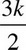• B.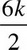• C.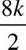• D.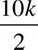13. The product ofb and a2 can be written as

• A. (ab)2
• B.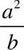• C. 2a ×b
• D.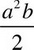14. xa = (x3)3

yb =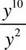What is the value of a × b ?

• A. 17
• B. 30
• C. 48
• D. 72

15. Hidden Glen Elementary school is collecting donations for a school charity drive. The total number of students in Mr. Greenwood's history class donate an average of y dollars each. The same number of students in Ms. Norris's science class donate an average of z dollars each. In terms of y and z, what is the average amount of donations for each student from both classes?

• A.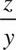• B.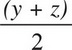• C. (y + z)
• D. 2(y + z)

16. What is the greatest common factor of (3xy)3 and 3x2y5 ?

• A. xy
• B. 3x2y5
• C. 3x2y3
• D. 27x3y3